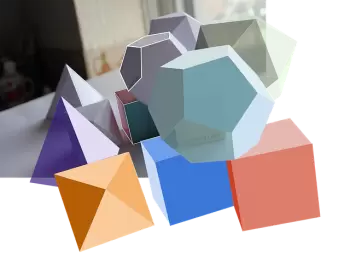# What Is a Polyhedron? Classification, Types and ExamplesA polyhedron definition is a 3D-solid shape limited only by a finite number of flat-faced geometric figures enclosing a fixed volume. The word polyhedron comes from the classical Greek πολύεδρον ( polyhedron ), with “poly” meaning many and “hedron” meaning surface.

These are the three parts of a polyhedron:

• Face: the flat surfaces that make up a polyhedron. These faces are polygons.

• Edge: the line segment shared by two flat surfaces.

• Vertex or corner: It is the intersection point of the different edges of the polyhedron.

In 1750, Leonhard Euler wrote his theorem for polyhedrons. The theorem indicates the relationship between the number of faces, the number of vertices (corner points), and the number of edges of a convex polyhedron.

Moreover, The famous Euler's formula expresses a constant not altered in rotations, translations of said polyhedrons. He concludes that there can only be five regular solids and establishes several relations in the proposition.

## What Are the Different Types of Polyhedrons?

The classification can be made according to the number of faces, edges, and vertices and their mutual relationships.

Depending on the features, the following types can be differentiated:

• A convex polyhedron is a geometric body whose surface can only be cut by a straight line at two points.

• In a concave polyhedron, a straight line can intersect its faces at more than two points, so it has some entering dihedral angle.

• A regular polyhedron is a solid whose faces are congruent regular polygons, and the number of faces that meet at each vertex is the same.

• An irregular polyhedron has unequal faces or angles. Among them are Archimedean solids and prisms and antiprisms.

• With uniform faces: all the faces are identical.

• With uniform edges: all its edges meet the same pair of polyhedron faces.

• With uniform vertex: all vertices meet the same number of faces and in the same order.

Platonic solids, regular or perfect, are convex polyhedra such that all their faces are regular polygons equal to each other, and in which all solid angles are equal.

## Examples of Polyhedra

Here are some examples in which these 3D figures appear in our daily life:

• Pyramids. Consisting of a base and various triangular faces such as the pyramids of Egypt. All vertices of the base are connected to the same point of intersection.

• Cubes. These shapes are made up of six identical squares. This geometric shape appears on the six-sided dice of a board game.

• Structural elements such as beams with a square base. This element is a parallelepiped since it is a solid shape formed by two regular squares and four equal rectangles.

• Soccer balls are made by joining 12 pentagons and 20 hexagons.

• Bees build their honeycombs in the shape of hexagonal prisms.Author:

Published: February 7, 2022
Last review: February 7, 2022